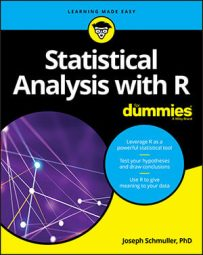##### Statistical Analysis with R For DummiesPerhaps the fundamental descriptive statistic is the number of scores in a set of data. `length() `is the R function that calculates this number. Work with the `Cars93` data frame, which is in the MASS package. (Click the check box next to MASS on the Packages tab.)

`Cars93` holds data on 27 variables for 93 cars available in 1993. What happens when you apply `length() `to the data frame?

```> length(Cars93) ``` ``` 27 ``` So `length()` returns the number of variables in the data frame. The function `ncol() `does the same thing:

```> ncol(Cars93) ``` ``` 27 ``` You already know the number of cases (rows) in the data frame, but if you had to find that number, `nrow() `would get it done:

```> nrow(Cars93) ``` ` 93`

If you want to know how many cases in the data frame meet a particular condition — like how many cars originated in the USA — you have to take into account the way R treats conditions: R attaches the label "TRUE" to cases that meet a condition, and "FALSE" to cases that don't. Also, R assigns the value `1` to "TRUE" and 0 to "FALSE."

To count the number of USA-originated cars, then, you state the condition and then add up all the 1s:

`> sum(Cars93\$Origin == "USA")`

` 48`

To count the number of non-USA cars in the data frame, you can change the condition to `"non-USA"`, of course, or you can use `!= —` the "not equal to" operator:

```> sum(Cars93\$Origin != "USA") ``` ``` 45 ``` More complex conditions are possible. For the number of 4-cylinder USA cars:

```> sum(Cars93\$Origin == "USA" & Cars93\$Cylinders == 4) ``` ``` 22 ```

Or, if you prefer no `\$`-signs:

```> with(Cars93, sum(Origin == "USA" & Cylinders == 4)) ``` ``` 22 ``` To calculate the number of elements in a vector, `length()`, as you may have read earlier, is the function to use. Here is a vector of horsepowers for 4-cylinder USA cars:

```> Horsepower.USA.Four <- Cars93\$Horsepower[Origin == ``````"USA" & Cylinders == 4] ``` and here's the number of horsepower values in that vector:

`> length(Horsepower.USA.Four)`

``` 22 ```